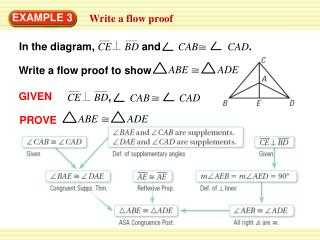DownloadDownload PresentationEXAMPLE 3

# EXAMPLE 3

Télécharger la présentation## EXAMPLE 3

- - - - - - - - - - - - - - - - - - - - - - - - - - - E N D - - - - - - - - - - - - - - - - - - - - - - - - - - -
##### Presentation Transcript

1. GIVEN CE BD, ∠ CAB CAD ABEADE PROVE EXAMPLE 3 Write a flow proof In the diagram,CE BDand∠ CAB CAD. ABEADE Write a flow proof to show

2. EXAMPLE 4 Standardized Test Practice

3. The locations of tower A, tower B, and the fire form a triangle. The dispatcher knows the distance from tower A to tower Band the measures of Aand B. So, the measures of two angles and an included side of the triangle are known. EXAMPLE 4 Standardized Test Practice By the ASA Congruence Postulate, all triangles with these measures are congruent. So, the triangle formed is unique and the fire location is given by the third vertex. Two lookouts are needed to locate the fire.

4. EXAMPLE 4 Standardized Test Practice ANSWER The correct answer is B.

5. STATEMENTS REASONS ABE ADE Given AEB AED Definition of right triangle Both are right angle triangle. ABE ADE Reflexive Property of Congruence AAS Congruence Theorem BD DB for Examples 3 and 4 GUIDED PRACTICE In Example 3, suppose ABEADEis also given. What theorem or postulate besides ASA can you use to prove that ABEADE? SOLUTION

6. What If?In Example 4, suppose a fire occurs directly between tower Band tower C. Could towers Band Cbe used to locate the fire? Explain The locations of tower B, tower C, and the fire form a triangle. The dispatcher knows the distance from tower B to tower Cand the measures of Band C. So, he knows the measures of two angles and an included side of the triangle. for Examples 3 and 4 GUIDED PRACTICE SOLUTION Proved by ASA congruence

7. for Examples 3 and 4 GUIDED PRACTICE By the ASA Congruence Postulate, all triangles with these measures are congruent. No triangle is formed by the location of the fire and tower, so the fire could be anywhere between tower B and C.